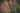In this series of articles, We've walked through overviews of Data Structures such as Linked List, Stack, Queue, Binary Search Tree, Binary Heap, and Hash Table. We also considered which one can be suitable to store vocabulary data as an example, and found Hash Table is the most sensible in the situation so far.

(Here is the course where I learned all these algorithms and data structures: JavaScript Algorithms and Data Structures Masterclass by Colt Steele - Udemy)

The data is used for my Chrome Extension project, and it structured like this at the moment:

// Result of console.log(MainData)
arbitrary: { category: "Book1", definition: "based on random choice or personal whim, rather than any reason or system.", tag: ["adj"]};
interpretation: { category: "Machine Learning", definition: "the action of explaining the meaning of something", tag:["noun"]};
intuitive: { category: "Book2", definition: "using or based on what one feels to be true even without conscious reasoning; instinctive", tag: ["adj"]};
precision: { category: "Machine Learning", definition: "the quality, condition, or fact of being exact and acurate", tag: ["noun"]};

Each vocabulary is a unique string, so that we used the word as index. In this structure, deleting/editing/inserting costs time complexity of O(1).

However, what if we implement Graph to the data instead of Hash Table? Does it cost as cheap as it is now, or help improve the function anyhow? In this article, we are going to study them.

Firstly, What is Graph?

Graph is a very common, widely used data structure. All Graphs always have two types of elements -- vertices and edges, and these make our Graph unique.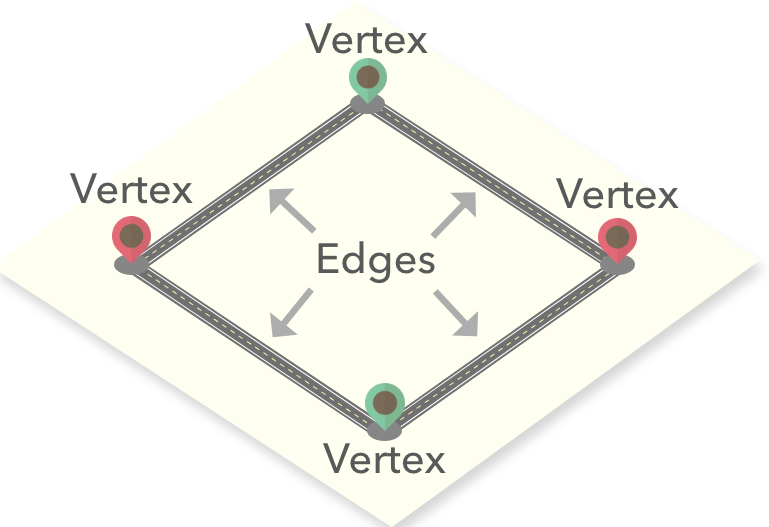As we see in the image above, vertices are the same as nodes which is a box that stores data into. edges are connections that connect vertices.

Two types of Graphs

There are two types of Graphs -- Directed Graph and Undirected Graph. For example, we can explain Instagram or Twitter relationship as Directed Graph, because there are directions between the relationship. When you follow someone, you make a connection to be able to see their content on your timeline, but they don't see your content as long as they don't follow you back -- to create a directed edge towards you.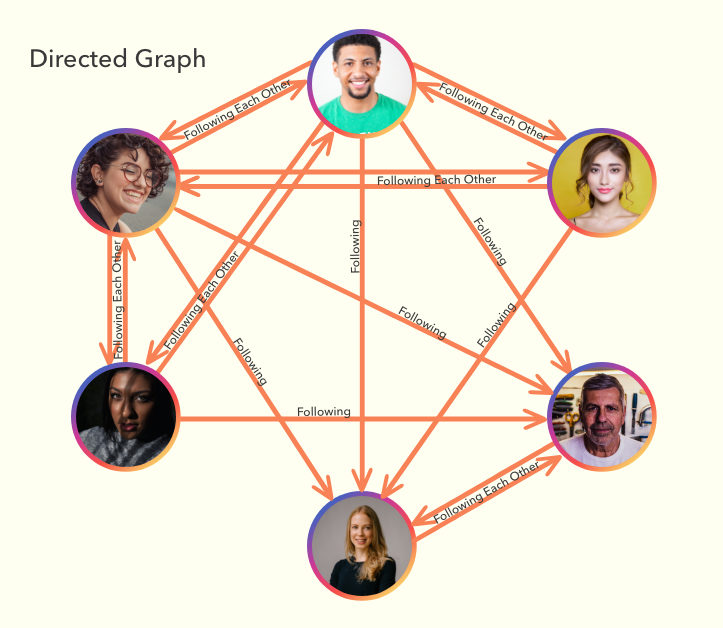Unlike Directed Graph, Undirected graph is used if directions don't need to be represented such as Facebook relationship. When you create an edge(accepting friend request), both you and the friend will be able to see each other's content automatically. Therefore there is no need to represent the direction.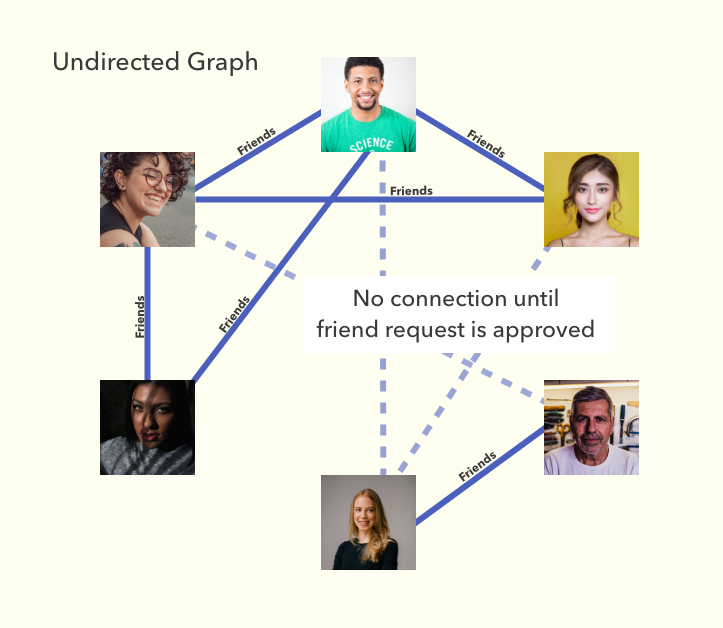Weighted/Unweighted Graph

One more useful thing about the Graph is that we can assign a **value** as its **weight/distance** on each edge. We call these Graphs **Weighted Graph**.

For example, if we decided to plot flight connections, we can use a Weighted Graph. We can assign a number to the edge between the connected airport, so that we can express the distance between them.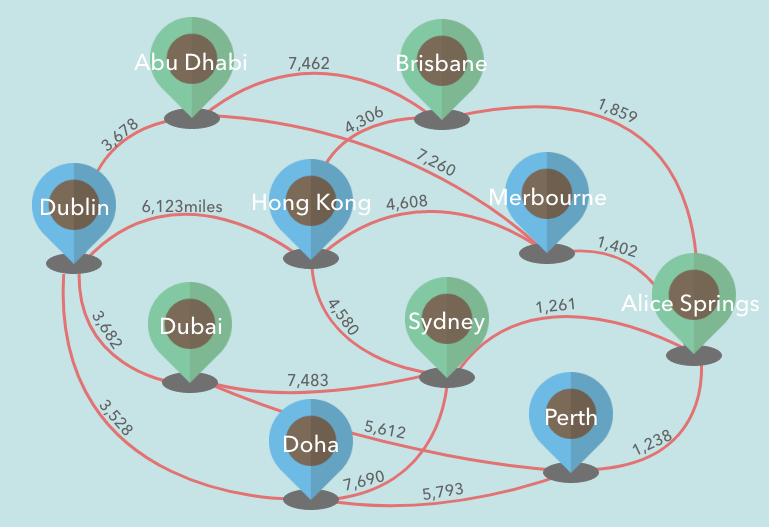How do we implement a Graph with JavaScript?

There are several different ways to implement it, such as Adjacency Matrix, Incidence Matrix, etc. Today we are going to look at one of the most common ways -- Adjacency List.

To represent an Adjacency list with JavaScript, we can use a key-value pair Hash Table. Each key-value pair describes the set of neighbors of a vertex in the graph.

Let's say we want to express flight connections with a Graph. Using a Hash Table to plot it, we can store the name of airports as keys. We can nest another Hash Table inside their value, and use destination as the key and distance/ (or cost) of the flight as the value.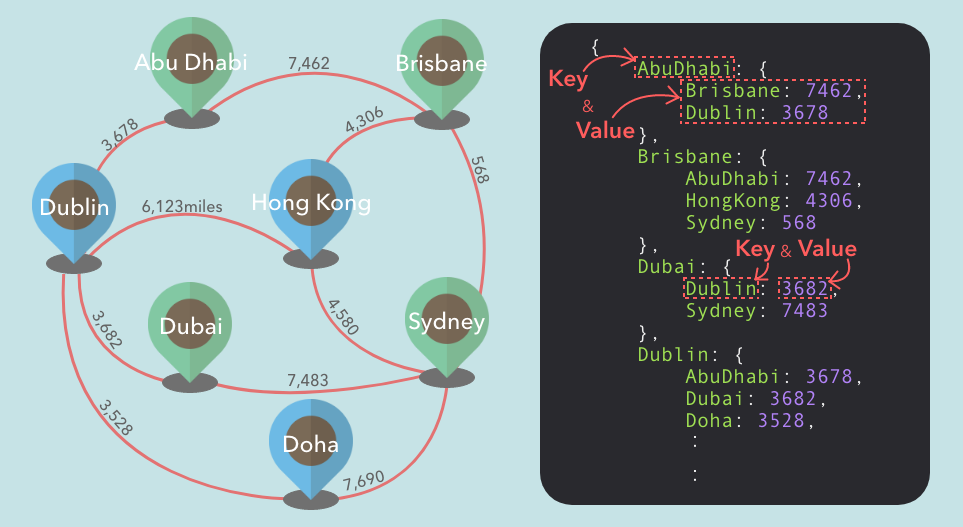Basic Implementation

So now, let's dive into coding! Firstly, we are going to create WeightGraph class to initialize a new object.

class WeightedGraph {
constructor() {
}
}
}
removeEdge() {
}
removeVertex() {
}
DFS() {
}
BFS() {
}
Dijkstra() {
}
}

Then, implement addVertex method for creating vertex without any connection, and addEdge method for creating an undirected edge between two vertices.

constructor() {
}
}
}
}

If we wanted directed edges with this addEdge method, we just need to remove the last line this.adjacencyList[vert2][vert1] = duration;.

The adjacency list and each vertex are all Hash tables, so that adding vertex/edges take time complexity of O(1).

Removing Edge and Vertex

In an Undirected Graph, an edge is assigned from two sides of vertices. Therefore if we want to remove a single edge completely, we need to delete them from both sides.

removeEdge(v1,v2) {
}

When we remove a vertex from our Graph, we want to make sure to delete edges that are connected to the removed vertex. We can use our removeEdge function to do this.

removeVertex(vert) {
for (let i in this.adjacencyList[vert]) {
this.removeEdge(vert, i);
}
}

Removing edge takes O(1) constant time. However, removing vertex takes O(|E|) which means it is subject to the length of its edges.

Traversal (Visiting Each Vertex)

Now we are going to create functions to traverse a graph. Our objective is to visit all the vertices one by one, but in Graph traversal, it may require to visit some vertices more than once. To revisit vertices as infrequently as possible, it is necessary to keep a record of which vertices have already been visited.

There are basically two algorithms for traversing a graph -- Depth-First Search and Breadth-First Search.

Depth-First Search

With DFS(shorthand for Depth-First Search), we visit the neighbor(child) vertex before visiting sibling vertex. So if we place the starting vertex on the top of the graph, we go towards straight to the bottom of the graph.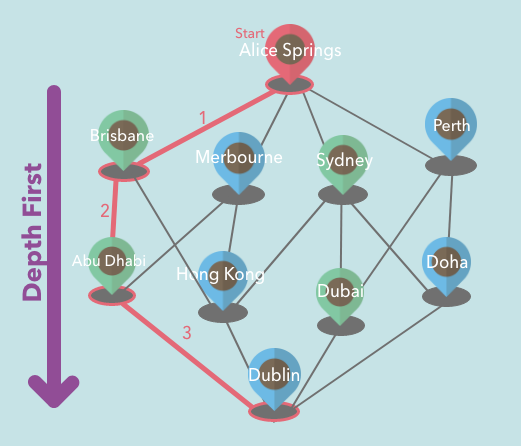Implementation:

DFS(target) {
const result = [];
const visited = {};
const helper = (vert) => {
if (!vert) return null;
visited[vert] = true;
result.push(vert);
for (let neighbor in this.adjacencyList[vert]) {
if (!visited[neighbor]) {
return helper(neighbor)
}
}
}
helper(target);
return result;
}

We have recursion in the helper function. If a neighbor of the target is not in the visited list, visit the neighbor and assign it as the target. Do the same to its neighbor and keep doing the same until there are no neighbors left to be added to the visited list.

With BFS(Breadth-First Search), we visit the sibling vertex before visiting neighbor(child) vertex. So if we are starting from the vertex on the top of the graph, we first go through all the neighbors of the starting vertex.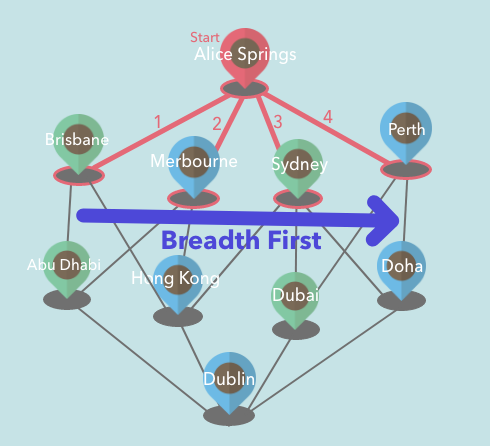Implementation:

BFS(start) {
const queue = [start];
const result = [];
const visited = {};
while(queue.length) {
let current = queue.shift();
visited[current] = true;
result.push(current)
for (let neighbor in this.adjacencyList[current]) {
if (!visited[neighbor]) {
visited[neighbor] = true;
queue.push(neighbor);
}
}
}
return result;
}

While using the same visited list as DFS, we also keep a record of where to visit the next in 'queue' array.

Finding the Shortest Path(Dijkstra Algorithm)

We will come across many occasions that we want to find out the shortest path from one to another in a graph.

Let's say we created an online travel agency, and we have a graph of cities with our special priced flights between those cities. What we want is to offer users the cheapest route from their city to their desired destination. However, without any function to calculate the cheapest route, we need to manually extract all the possible routes and compare each other -- which would be time-consuming and hurt our neck.

Dijkstra's Algorithm is the way Edsger W. Dijkstra conceived to solve this problem 64 years ago.How Dijkstra's Algorithm Works

We will need three storage to keep track of the main information:

1. A list of all the airports, and its total cost from starting airport.
2. A list that tells you which route has the total cheapest cost so far -- and this also tells you which airport we should visit next.
3. A list of all the airports, and the record of which airport we previously visited to reach the airport.

Basically that's all we need to keep a record of, and all of them are updated as we go through the algorithm.

Initiallization

Let's say we are going to find the cheapest route from Dublin to Alice Springs. So we can assign flight costs as the weight of edges.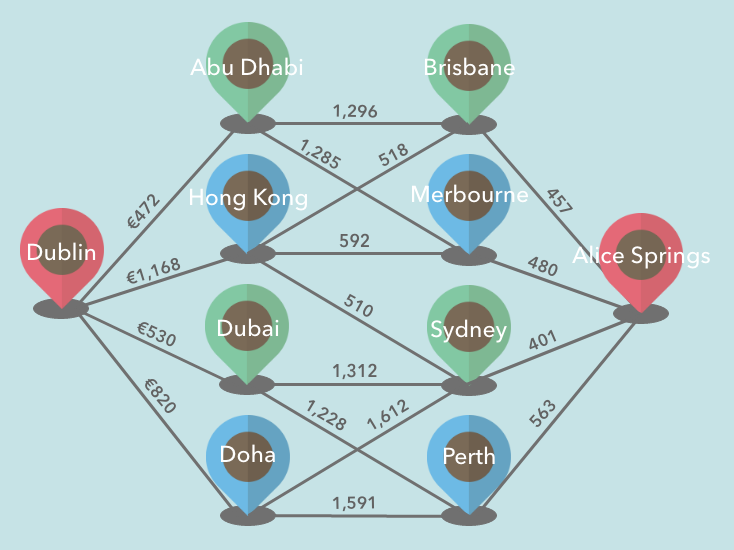And we plot it with our Graph.

// console.log
{
AbuDhabi: {
Brisbane: 1296,
Melbourne: 1285
},
AliceSprings: {
Brisbane: 457,
Melbourne: 480,
Perth: 563,
Sydney: 401
},
Brisbane: {
AbuDhabi: 1296,
HongKong: 518
},
.
.
.
Sydney: {
AliceSprings: 401,
Dubai: 1312,
Doha: 1612,
HongKong: 510
}
}

We don't know any information to assign the lists yet, except the total cost of Dublin to Dublin which is zero. And the rest of the airports, we are going to assign Infinity so that whenever we discover new costs, it will be cheaper than the initialization.

Now we can assign List2 which tells you the route that has the cheapest cost -- because we assigned zero to the route Dublin to Dublin, which is the cheapest so far.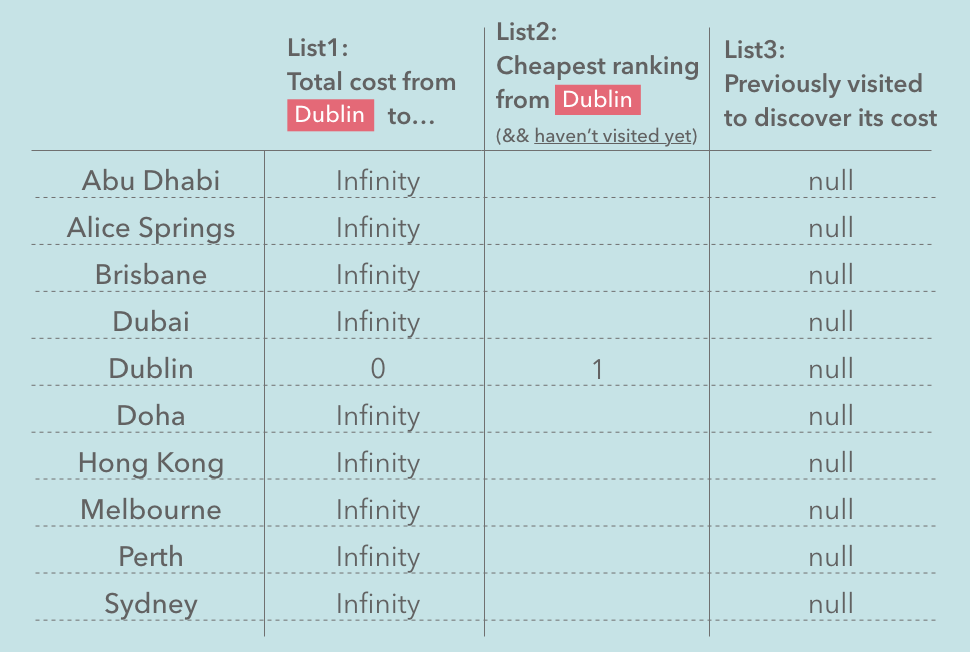Initiallization in code

Now let's initialize these lists on code. Firstly, we'll create our Priority Queue class for organizing the List2 -- The list that tells you which route has the total cheapest cost at the moment.

class PriorityQueue {
constructor(){
this.values = [];
}
enqueue(val, priority) {
this.values.push({val, priority});
this.sort();
};
dequeue() {
return this.values.shift();
};
sort() {
this.values.sort((a, b) => a.priority - b.priority);
};
}

The lowest number assigned in priority will come to the beginning of the queue.

Next, we create Dijkstra's algorithm function that accepts starting vertex and the last stop vertex.

Dijkstras(start, finish) {
// List1
const costFromStartTo = {};
// List2
const checkList = new PriorityQueue();
// List3
const prev = {};

let current;
let result = [];
for (let vert in this.adjacencyList) {

}
while (checkList.values.length) {

}
}

Inside, we create three lists to keep records on.

• List1 is to keep all the vertices with numbers that represent its total cost from starting vertex. We name it costFromStartTo.
• List2 is the priority queue we implemented earlier. we call it checkList -- because this queue tells you which vertex needs to be checked next.
• List3 is a list of all the vertices that keep the record of which vertex was previously visited to discover its current cost. So that we call it prev.

shortest and result will be used inside while loop later on.

Inside for loop, we'll fill the lists with zero and Infinity which is all we know about the starting point and the rest of the vertices.

let current;
let result = [];
for (let vert in this.adjacencyList) {
if (vert === start) {
costFromStartTo[vert] = 0;
checkList.enqueue(vert, 0);
} else {
costFromStartTo[vert] = Infinity;
}
prev[vert] = null;
}

If we run Dijkstras("Dublin", "AliceSprings"); now, all the lists should be filled like this: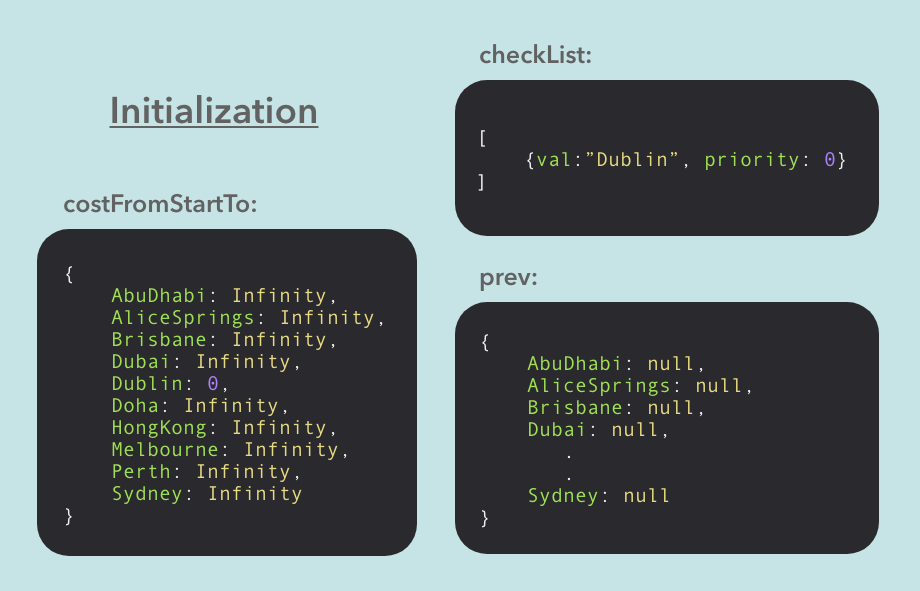Calculate to update `costFromStartTo` list

What basically we want to do is, keep calculating to update costFromStartTo list. As we already calculated the cost from the start to the same start, we can look at the vertices that are neighbors to the starting vertex. Now we can calculate their total cost from the starting vertex.

To do this on code:

for (let vert in this.adjacencyList) {
.
.
.
}
while (checkList.values.length) {
current = checkList.dequeue().val;
for (let neighbor in this.adjacencyList[current]) {

}
}

We choose to check vertices that are neighbors to the vertex that currently holds the cheapest total cost.

To find out a vertex with the cheapest total cost, we can simply look at the first vertex in the checkList. At the same time, we can remove it from the list, so the vertex won't be visited again as long as it won't be updated with a new cheaper route.

Then, we can loop over each connected vertex and update the three lists as we calculate each cost.

while (checkList.values.length) {
current = checkList.dequeue().val;
for (let neighbor in this.adjacencyList[current]) {
let costToNeighbor = costFromStartTo[current] + this.adjacencyList[current][neighbor];
if (costToNeighbor < costFromStartTo[neighbor]) {
costFromStartTo[neighbor] = costToNeighbor;
prev[neighbor] = current;
checkList.enqueue(neighbor, costToNeighbor);
}
}
}

We add up the cost from start to the current vertex, and the individual cost from the current vertex to the neighbor. If the sum is cheaper than the current cost on the list costFromStartTo for the neighbor, we update the list with the sum.

We also update prev[neighbor] = current to remember which route was the cheapest to get the neighbor.

At this point, we also need to add the neighbor to the CheckList. After assigning all the neighbors in the CheckList, you know which neighbor is at the moment cheapest. And it also means it holds the highest possibility to get to the last stop at the moment.

Now, we just need to loop over this procedure until we hit the last stop at the beginning of the priority queue visitedList.

while (checkList.values.length) {
current = checkList.dequeue().val;
if (current === finish) {
// Done
while (prev[current]) {
result.push(current);
current = prev[current];
}
break;
}
else {
for (let neighbor in this.adjacencyList[current]) {
let costToNeighbor = costFromStartTo[current] + this.adjacencyList[current][neighbor];
if (costToNeighbor < costFromStartTo[neighbor]) {
costFromStartTo[neighbor] = costToNeighbor;
prev[neighbor] = current;
checkList.enqueue(neighbor, costToNeighbor);
}
}
}
}
return result.concat(current).reverse();

When we extract the last stop from the checkList, we can stop all the procedure -- so we create if statement to finish the loop, and wrap the procedure to update the list with else statement.

In the end, we reverse the result list and return it.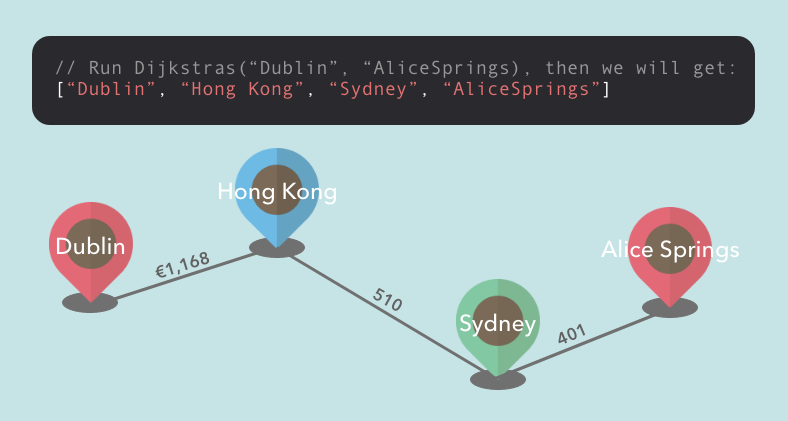Conclusion

If we want to represent complicated connections between data, Graph can be a suitable Data Structure for us. In other words, we don't need to use Graph if there is no connection between nodes to influence decision making. Therefore, going back to the first question -- Do we want to implement Graph to organize a vocabulary list? Probably the best is to stick with a simple Hash Table, because we don't present certain connections between vocabularies.

Thank you so much for reading! Please leave a comment if you have any thoughts, or ideas to improve the code, I would be so grateful to hear from you. :)

Resources

JavaScript Algorithms and Data Structures Masterclass by Colt Steele - Udemy

Graph (abstract data type) - Wikipedia